# NCERT Solutions class 7 Maths Chapter-6 Exercise 6.3

NCERT Solutions Class-7 Maths chapter-6 Triangle and its properties Exercise-6.3 is prepared by academic team of pw all the questions of NCERT text book are solved step by step with proper and detail solutions explaining each and every questions . For More and additional questions of CBSE class 7 maths you can go to class 7 maths sections. NCERT class 7 Maths Solutions is the best way to enhanced your mathematics skill. And pw practice worksheet & question bank will help you a lot .

## NCERT Solutions class 7 Maths Chapter-6 Triangle and its properties

### Solutions of Chapter Triangle and its properties Exercise-6.3

Question 1:

Find the value of the unknown x in the following diagrams: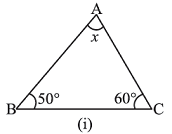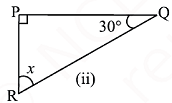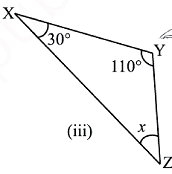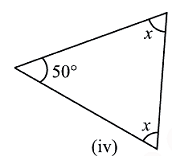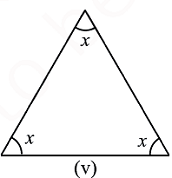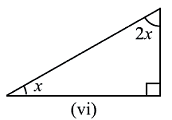(i) In the above question, we have

1st interior angle = x, 2nd interior angle = 50o and 3rd interior angle = 60o

We have to find out the value of x

Thus,

x + 50o + 60o = 180o  (angle sum property of triangle)

x + 110o = 180o

x = 180o – 110o

x = 70o

Hence, the value of x is 70o

(ii) In the above question, we have

1st interior angle = x, 2nd interior angle = 90o and 3rd interior angle = 30o

We have to find out the value of x

Thus,

x + 90o + 30o = 180o   (angle sum property of triangle)

x + 120o = 180o

x = 180o – 120o

x = 60o

Hence, the value of x is 60o

(iii) In the above question, we have

1st interior angle = x, 2nd interior angle = 30o and 3rd interior angle = 110o

We have to find out the value of x

Thus,

x + 30o + 110o = 180o (angle sum property of triangle)

x + 140o = 180o

x = 180o – 140o

x = 40o

Hence, the value of x is 40o

(iv) In the above question, we have

1st interior angle = 50o, 2nd interior angle = x and 3rd interior angle = x

We have to find out the value of x

Thus,

50o + x + x = 180o    (angle sum property of triangle)

2x + 50o = 180o

2x = 180o – 50o

2x = 130o

x = 130/2

x = 65o

Hence, the value of x is 65o

(v) In the above question, we have

1st interior angle = x, 2nd interior angle = x and 3rd interior angle = x

We have to find out the value of x

And,

x + x + x = 180o     (angle sum property of triangle)

3x = 180o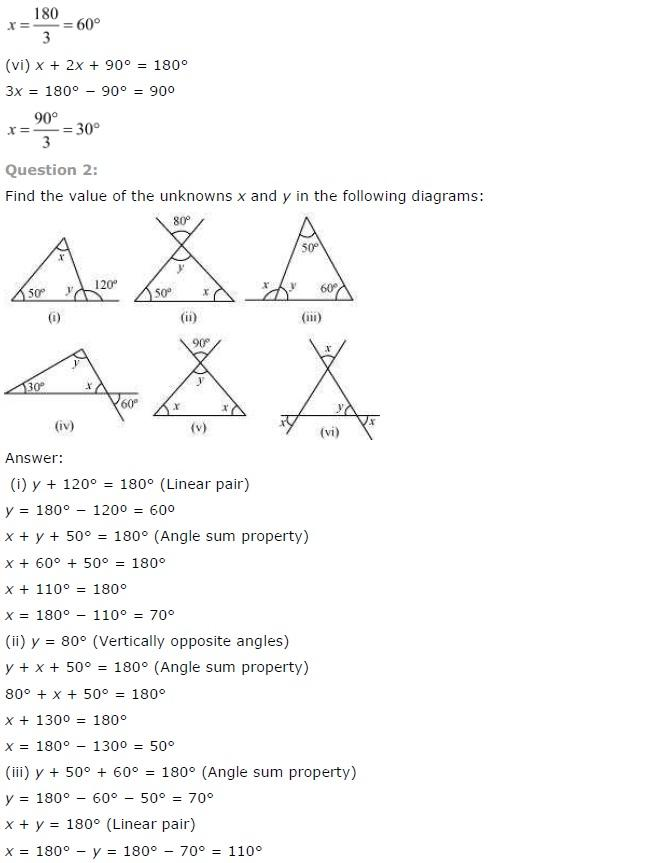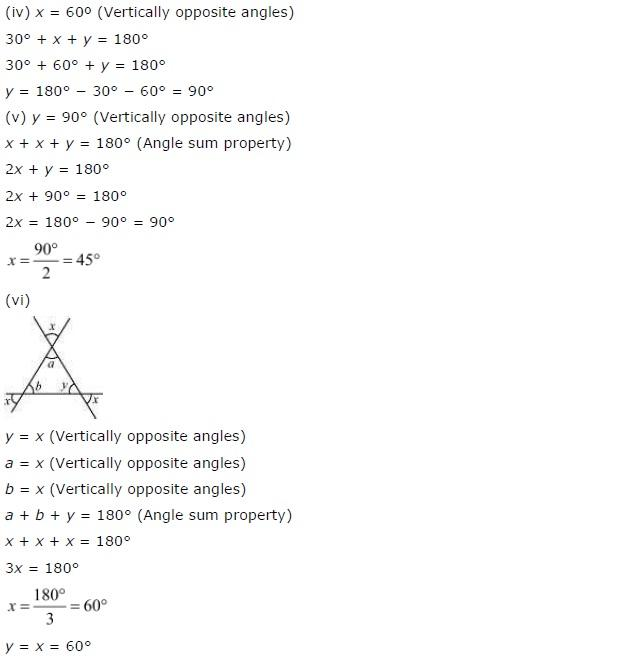NCERT CLASS 7 MATHEMATICS SOLUTIONS